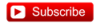# YouTubeT4-40: Fixed Income: Bullet versus Barbell Bond Portfolio

#### Nicole Seaman

##### Director of FRM Operations
Staff member
Subscriber
The bullet portfolio invests in a single medium-term bond. The corresponding barbell portfolio invests the same amount of capital and achieves the same duration, but invests in a mix of the short-term plus long-term bond. But the barbell portfolio will have greater convexity. Tuckman explains: "The barbell has greater convexity than the bullet because duration increases linearly with maturity while convexity increases with the square of maturity. If a combination of short and long durations, essentially maturities, equals the duration of the bullet, that same combination of the two convexities, essentially maturities squared, must be greater than the convexity of the bullet."#### David Harper CFA FRM

##### David Harper CFA FRM
Staff member
Subscriber
HI @MAli9983 On YouTube, I pinned my comment that explains those calculations:
In the video, I said I would explain how I solved for the amount that should be invested in the short-term bond in order to achieve a duration with the barbell portfolio that matches the duration of the bullet portfolio. The key is to match dollar durations because if the dollar durations match, then the durations match (given the investment dollars are the same). Dollar duration equals investment multiplied by modified duration; that is, let D = modified duration and let V = value of dollars invested, such that DV = dollar duration. Further, define S = duration of short-term bond; M = duration of (bullet) medium-term bond; and L = duration of long-term bond. The dollar duration, then, of the bullet portfolio is simply V*M or, in my example, 63.763*15 = 956.44 million. The dollar duration of the barbell portfolio is given by S*V(small) + L*V(large), where V(small) and V(large) are the respective dollar investments. However, we require that V(small) + V(large) = V, and V(large) = V - V(small) such that the dollar duration of the barbell is given by S*V(small) + L*[V - V(small)]. Then the equality is given by: S*V(small) + L*[V - V(small)] = M*V --> S*V(small) + L*V - L*V(small)] = M*V --> V(small)*(S-L) + L*V = M*V --> V(small)*(S-L) = M*V - L*V --> V(small) = (M*V - L*V)/(S-L). In my example, S = 5.0, M = 15.0 and L = 30.0 such that V(small) = (15.0*V - 30*V)/(5.0 - 30.0) is the solution for V(small) as a function of V. In this case, V(small) = (15.0*63.763 - 30*63.763)/(5.0 - 30.0) = 38.26 million to the 5-year bond and the remaining 25.51 to the 30-year bond which turns out to be 60/40%. Thanks!
... then, of course, once we've solved for 38.26 we can see that $63.763 -$38.26 = \$25.51. I hope that's helpful!